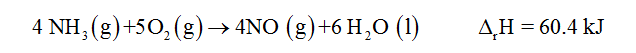# When 3.52 g ammonia gas is oxidized over a platinum catalyst to form nitrogen monoxide gas and water, 60.4 kJ of heat are released. Develop a complete thermochemical equation for this process, using smallest possible coefficients. Then calculate the enthalpy change when 2 moles of nitrogen monoxide react with water to form ammonia and 2.5 moles of oxygen.thank you I really do not understand this

Question
1 views

When 3.52 g ammonia gas is oxidized over a platinum catalyst to form nitrogen monoxide gas and water, 60.4 kJ of heat are released. Develop a complete thermochemical equation for this process, using smallest possible coefficients. Then calculate the enthalpy change when 2 moles of nitrogen monoxide react with water to form ammonia and 2.5 moles of oxygen.

thank you I really do not understand this

check_circle

Step 1

The thermochemical equation for the given reaction is given below:...

### Want to see the full answer?

See Solution

#### Want to see this answer and more?

Solutions are written by subject experts who are available 24/7. Questions are typically answered within 1 hour.*

See Solution
*Response times may vary by subject and question.
Tagged in